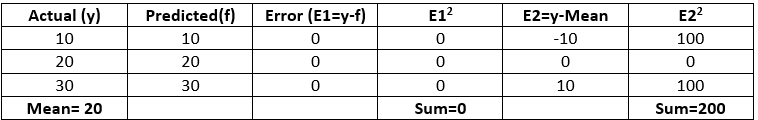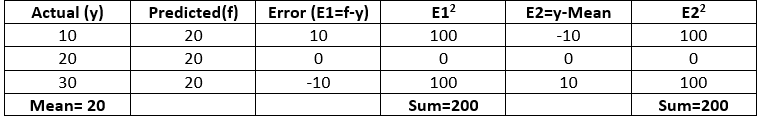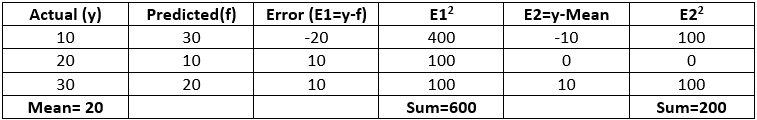# Python – Coefficient of Determination-R2 score

Coefficient of determination also called as R2 score is used to evaluate the performance of a linear regression model. It is the amount of the variation in the output dependent attribute which is predictable from the input independent variable(s). It is used to check how well-observed results are reproduced by the model, depending on the ratio of total deviation of results described by the model.

Mathematical Formula:

`R2= 1- SSres / SStot`

Where,
SSres is the sum of squares of the residual errors.
SStot is the total sum of the errors.

Interpretation of R2 score:
Assume R2 = 0.68
It can be referred that 68% of the changeability of the dependent output attribute can be explained by the model while the remaining 32 % of the variability is still unaccounted for.
R2 indicates the proportion of data points which lie within the line created by the regression equation. A higher value of R2 is desirable as it indicates better results.

Examples
Case 1 Model gives accurate results`  R2 = 1- 0/200 = 1`

Case 2 Model gives same results always` R2 = 1- 200/200 = 0`

Case 3 Model gives ambiguous results` R2 = 1- 600/200 = -2`

We can import r2_score from sklearn.metrics in Python to compute R2 score.

Python Implementation:
Code 1: Import r2_score from sklearn.metrics

 `from` `sklearn.metrics ``import` `r2_score `

Code 2: Calculate R2 score for all the above cases.

 `### Assume y is the actual value and f is the predicted values ` `y ``=``[``10``, ``20``, ``30``] ` `f ``=``[``10``, ``20``, ``30``] ` `r2 ``=` `r2_score(y, f) ` `print``(``'r2 score for perfect model is'``, r2) `

Output:

`r2 score for perfect model is 1.0`

 `### Assume y is the actual value and f is the predicted values ` `y ``=``[``10``, ``20``, ``30``] ` `f ``=``[``20``, ``20``, ``20``] ` `r2 ``=` `r2_score(y, f) ` `print``(``'r2 score for a model which predicts mean value always is'``, r2) ` ` `

Output:

`r2 score for a model which predicts mean value always is 0.0`

Code 3:

 `### Assume y is the actual value and f is the predicted values ` `y ``=` `[``10``, ``20``, ``30``] ` `f ``=` `[``30``, ``10``, ``20``] ` `r2 ``=` `r2_score(y, f) ` `print``(``'r2 score for a worse model is'``, r2) `

Output:

`r2 score for a worse model is -2.0`

Conclusion:

• The best possible score is 1 which is obtained when the predicted values are the same as the actual values.
• R2 score of baseline model is 0.
• During the worse cases, R2 score can even be negative.

My Personal Notes arrow_drop_upCheck out this Author's contributed articles.

If you like GeeksforGeeks and would like to contribute, you can also write an article using contribute.geeksforgeeks.org or mail your article to contribute@geeksforgeeks.org. See your article appearing on the GeeksforGeeks main page and help other Geeks.

Please Improve this article if you find anything incorrect by clicking on the "Improve Article" button below.